edit

# Grove - Thermal Imaging Camera IR-Array MLX90641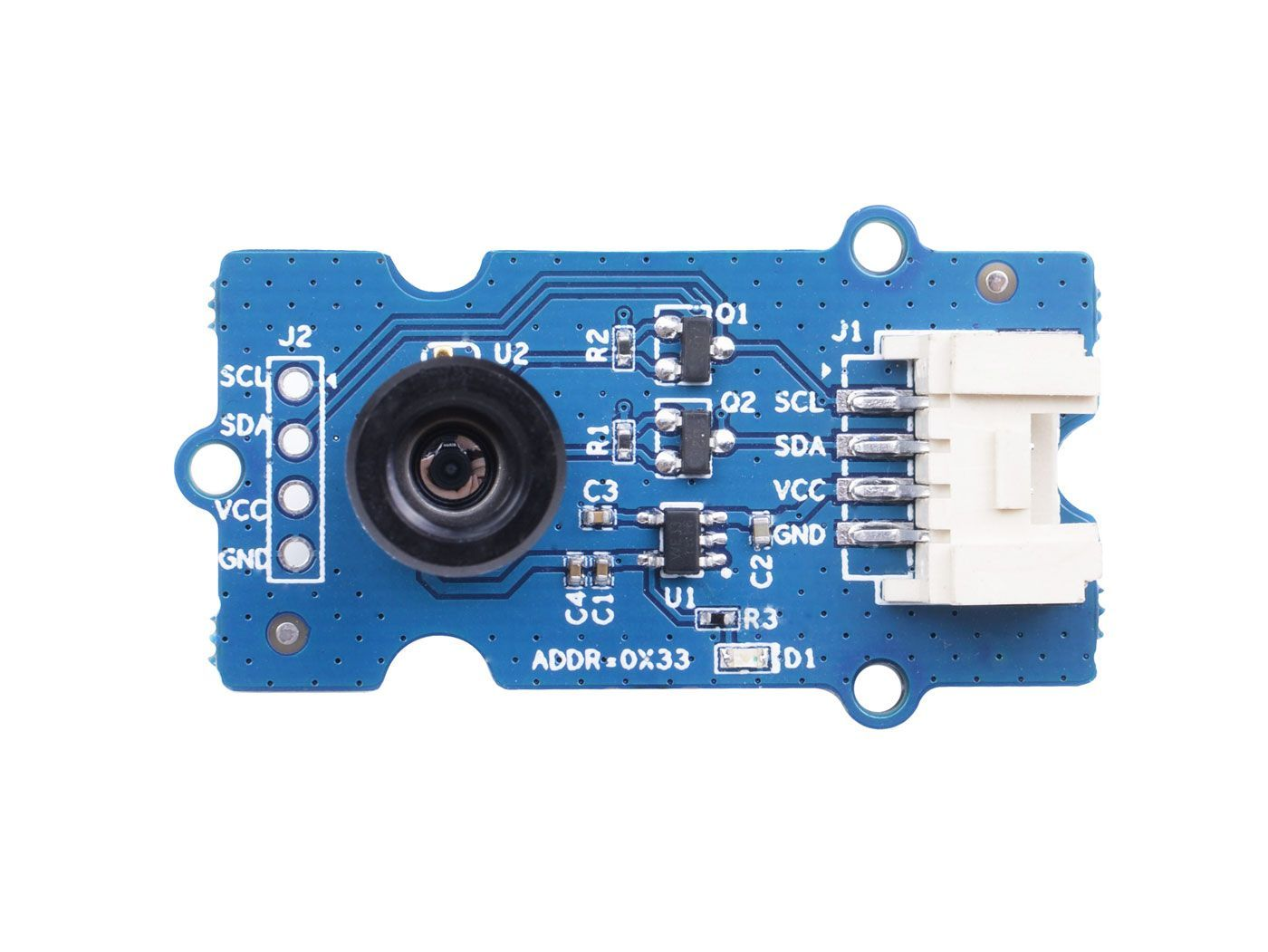This IR thermal camera carries a 16x12 array of thermal sensors (MLX90641) and it can detect the temperature of objects from far away with a center area accuracy of ±1℃ and average accuracy of ±1.5℃. In order to obtain the thermal images easily, the I2C protocol is used to get the low-resolution images from the camera. The FOV (Field of View) of this camera is 110°x75°, and the temperature measurement range is -40℃ to 300℃. In order to obtain the thermal image easily, I2C protocol is used to get the low-resolution image from the camera.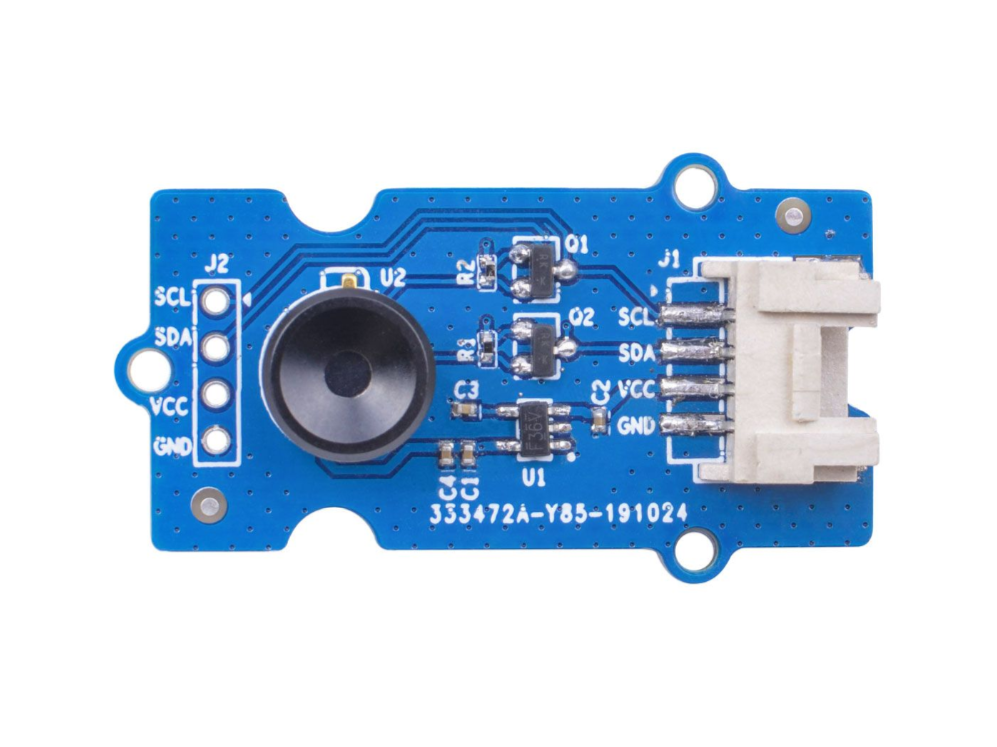While Grove - Thermal Imaging Camera is a thermal sensor (MLX90640) , carrying a 32x24 array of thermal sensors, and it can detect the temperature of objects from feet away with the accuracy of ±1.5℃ and can present dynamic thermal images and detect the surrounding temperature from -40℃~300℃. The camera with narrow-angle/wide-angle has an FOV(Field of View) of 55°x35°/110°x75°.In order to obtain the thermal image easily, I2C protocol is used to get the low-resolution image from the camera.

## Versions¶

Version Date of Released Order
Grove - Thermal Imaging Camera / IR Array MLX90641 110 degree [New] 03-June-2020 Buy it
Grove - Thermal Imaging Camera / IR Array MLX90640 110 degree 12-Nov-2019 Buy it

Note

This wiki fits both types of the Thermal Imageing Camera IR Array MLX90641 and MLX90640.

## Features¶

• Compact size 16x12 pixel IR thermal sensor array (MLX90641)，32x24 array pixel IR thermal sensor array (MLX90640)
• High FOV (field-of-view) of 110°x75° to capture more area
• Wide temperature measurement range (-40℃~300℃)
• I2C Grove interface for easy communication with an MCU
• Fully calibrated IR array for convenient setup

## Specification¶

Item Grove - Thermal Imaging Camera - MLX90640 Grove - Thermal Imaging Camera - MLX90641
Thermal sensor 32X24 array MLX90640 16x12 array MLX90641
Operating Voltage 3.3V - 5V 3.3V - 5V
Current consumption ~18mA ~18mA
FOV(Field of View) 110°x75° 110°x75°
Temperature Measurement Range -40°C - 300°C -40°C - 300°C
Temperature Resolution ± 1.5°C ± 1.5°C (±1℃ at center area)
Refresh Rate 0.5Hz - 64Hz 0.5Hz - 64Hz
Interface I2C Grove interface I2C Grove interface

## Platforms Supported¶

Arduino Raspberry Pi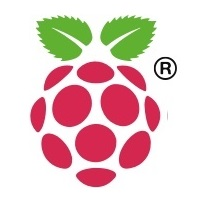## Getting Started¶

### Getting Started by Wio Terminal¶

Materials required

Wio Terminal Grove - Thermal Imaging Camera / IR Array MLX90641 110 degree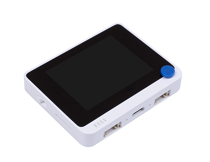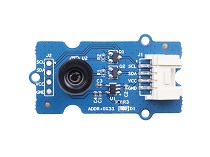Get one now Get one now

#### Hardware Connection¶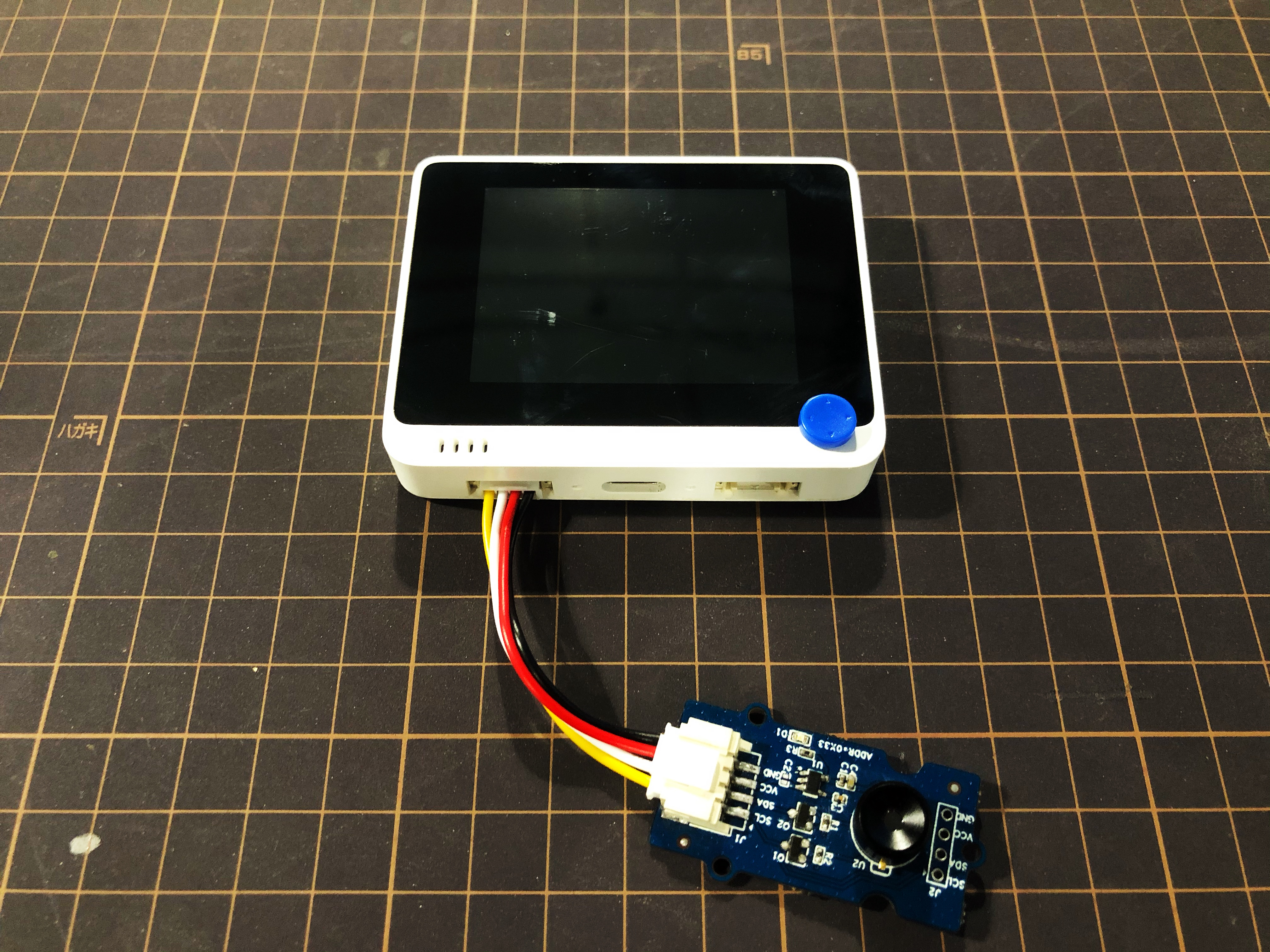Step 1. Plug Grove - Thermal Imaging Camera to Wio Terminal via Grove cable and also connect Wio Terminal to PC through a USB cable.

Step 2. Download the Library and copy the whole Seeed_Arduino_MLX9064x file and paste it into your Arduino IDE library file.

Note

If it is your first time playing Wio Terminal and not sure which interface to plug on Wio Terminal, please refer to Get Started with Wio Terminal.

Step 3. Copy the Software Code 1 below into your Arduino IDE and upload it for visualization format displayed via Serial Port.

#### Outcome of Visualization Format¶

Software Code 1

/*
Output the temperature readings to all pixels to be read by a Processing visualizer
*/

#include <Wire.h>

#define USE_MLX90641

#ifndef USE_MLX90641
#include "MLX90640_API.h"
#else
#include "MLX90641_API.h"
#endif

#include "MLX9064X_I2C_Driver.h"

#if defined(ARDUINO_ARCH_AVR)
#define debug  Serial

#elif defined(ARDUINO_ARCH_SAMD) ||  defined(ARDUINO_ARCH_SAM)
#define debug  Serial
#else
#define debug  Serial
#endif

#ifdef USE_MLX90641
const byte MLX90641_address = 0x33; //Default 7-bit unshifted address of the MLX90641
#define TA_SHIFT 8 //Default shift for MLX90641 in open air

uint16_t eeMLX90641;
float MLX90641To;
uint16_t MLX90641Frame;
paramsMLX90641 MLX90641;
int errorno = 0;
#else
const byte MLX90640_address = 0x33; //Default 7-bit unshifted address of the MLX90640

#define TA_SHIFT 8 //Default shift for MLX90640 in open air

float mlx90640To;
paramsMLX90640 mlx90640;
#endif
void setup() {
Wire.begin();
Wire.setClock(400000); //Increase I2C clock speed to 400kHz

debug.begin(115200); //Fast debug as possible

while (!debug); //Wait for user to open terminal
//debug.println("MLX90640 IR Array Example");

#ifndef USE_MLX90641
if (isConnected() == false) {
while (1);
}
//Get device parameters - We only have to do this once
int status;
uint16_t eeMLX90640;
if (status != 0) {
}

status = MLX90640_ExtractParameters(eeMLX90640, &mlx90640);
if (status != 0) {
debug.println("Parameter extraction failed");
}

//Once params are extracted, we can release eeMLX90640 array

//MLX90640_SetRefreshRate(MLX90640_address, 0x02); //Set rate to 2Hz
MLX90640_SetRefreshRate(MLX90640_address, 0x03); //Set rate to 4Hz
//MLX90640_SetRefreshRate(MLX90640_address, 0x07); //Set rate to 64H
#else
if (isConnected() == false) {
while (1);
}
//Get device parameters - We only have to do this once
int status;
errorno = status;//MLX90641_CheckEEPROMValid(eeMLX90641);//eeMLX90641 & 0x0040;//

if (status != 0) {
while(1);
}

status = MLX90641_ExtractParameters(eeMLX90641, &MLX90641);
//errorno = status;
if (status != 0) {
debug.println("Parameter extraction failed");
while(1);
}

//Once params are extracted, we can release eeMLX90641 array

//MLX90641_SetRefreshRate(MLX90641_address, 0x02); //Set rate to 2Hz
MLX90641_SetRefreshRate(MLX90641_address, 0x03); //Set rate to 4Hz
//MLX90641_SetRefreshRate(MLX90641_address, 0x07); //Set rate to 64Hz
#endif

}

void loop() {
#ifndef USE_MLX90641
long startTime = millis();
for (byte x = 0 ; x < 2 ; x++) {
uint16_t mlx90640Frame;

float vdd = MLX90640_GetVdd(mlx90640Frame, &mlx90640);
float Ta = MLX90640_GetTa(mlx90640Frame, &mlx90640);

float tr = Ta - TA_SHIFT; //Reflected temperature based on the sensor ambient temperature
float emissivity = 0.95;

MLX90640_CalculateTo(mlx90640Frame, &mlx90640, emissivity, tr, mlx90640To);
}
long stopTime = millis();

for (int x = 0 ; x < 768 ; x++) {
//if(x % 8 == 0) debug.println();
debug.print(mlx90640To[x], 2);
debug.print(",");
}
debug.println("");
#else
long startTime = millis();

for (byte x = 0 ; x < 2 ; x++) {

float vdd = MLX90641_GetVdd(MLX90641Frame, &MLX90641);
float Ta = MLX90641_GetTa(MLX90641Frame, &MLX90641);

float tr = Ta - TA_SHIFT; //Reflected temperature based on the sensor ambient temperature
float emissivity = 0.95;

MLX90641_CalculateTo(MLX90641Frame, &MLX90641, emissivity, tr, MLX90641To);
}
long stopTime = millis();
/*
debug.print("vdd=");
debug.print(vdd,2);
debug.print(",Ta=");
debug.print(Ta,2);

debug.print(",errorno=");
debug.print(errorno,DEC);

for (int x = 0 ; x < 64 ; x++) {
debug.print(MLX90641Frame[x], HEX);
debug.print(",");
}

delay(1000);
*/
for (int x = 0 ; x < 192 ; x++) {
debug.print(MLX90641To[x], 2);
debug.print(",");
}
debug.println("");
#endif
}

//Returns true if the MLX90640 is detected on the I2C bus
boolean isConnected() {
#ifndef USE_MLX90641
#else
#endif
if (Wire.endTransmission() != 0) {
return (false);    //Sensor did not ACK
}
return (true);
}


Note

Upload the software code 1 above into your Arduino IDE and open the Serial Port, you will see an outcome of visualization format as following: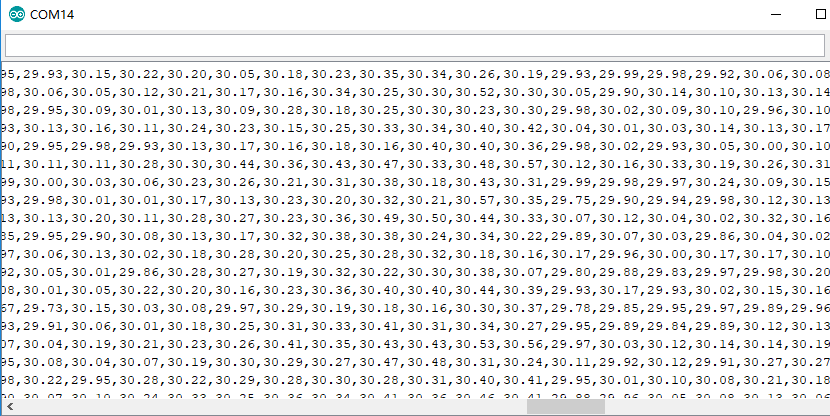#### Outcome of Visualization on Wio Terminal¶

Step 4. Upload the Software Code 2 below into your Arduino IDE for visualization displayed on Wio Terminal.

Software Code 2

#include <Wire.h>
#include "MLX90641_API.h"
#include "MLX9064X_I2C_Driver.h"
#include <TFT_eSPI.h>                // Include the graphics library (this includes the sprite functions)

const byte MLX90641_address = 0x33; //Default 7-bit unshifted address of the MLX90641
#define TA_SHIFT 12 //Default shift for MLX90641 in open air
#define debug  Serial
uint16_t eeMLX90641;
float MLX90641To;
uint16_t MLX90641Frame;
paramsMLX90641 MLX90641;
int errorno = 0;

TFT_eSPI    tft = TFT_eSPI();
TFT_eSprite Display = TFT_eSprite(&tft);  // Create Sprite object "img" with pointer to "tft" object
// the pointer is used by pushSprite() to push it onto the TFT

unsigned long CurTime;

uint16_t TheColor;
uint16_t MinTemp = 25;
uint16_t MaxTemp = 38;

// variables for interpolated colors
byte red, green, blue;

// variables for row/column interpolation
byte i, j, k, row, col, incr;
float intPoint, val, a, b, c, d, ii;
byte aLow, aHigh;

// size of a display "pixel"
byte BoxWidth = 3;
byte BoxHeight = 3;

int x, y;
char buf;

// variable to toggle the display grid
int ShowGrid = -1;

// array for the interpolated array
float HDTemp;

void setup() {
Wire.begin();
Wire.setClock(2000000); //Increase I2C clock speed to 2M
debug.begin(115200); //Fast debug as possible

// start the display and set the background to black

if (isConnected() == false) {
while (1);
}
//Get device parameters - We only have to do this once
int status;
errorno = status;//MLX90641_CheckEEPROMValid(eeMLX90641);//eeMLX90641 & 0x0040;//

if (status != 0) {
while(1);
}

status = MLX90641_ExtractParameters(eeMLX90641, &MLX90641);
//errorno = status;
if (status != 0) {
debug.println("Parameter extraction failed");
while(1);
}

//Once params are extracted, we can release eeMLX90641 array

MLX90641_SetRefreshRate(MLX90641_address, 0x05); //Set rate to 16Hz

tft.begin();
tft.setRotation(3);
tft.fillScreen(TFT_BLACK);
Display.createSprite(TFT_HEIGHT, TFT_WIDTH);
Display.fillSprite(TFT_BLACK);

// get the cutoff points for the color interpolation routines
// note this function called when the temp scale is changed
Getabcd();

// draw a legend with the scale that matches the sensors max and min
DrawLegend();
}
void loop() {
// draw a large white border for the temperature area
Display.fillRect(10, 10, 220, 220, TFT_WHITE);
for (byte x = 0 ; x < 2 ; x++) {

float vdd = MLX90641_GetVdd(MLX90641Frame, &MLX90641);
float Ta = MLX90641_GetTa(MLX90641Frame, &MLX90641);

float tr = Ta - TA_SHIFT; //Reflected temperature based on the sensor ambient temperature
float emissivity = 0.95;

MLX90641_CalculateTo(MLX90641Frame, &MLX90641, emissivity, tr, MLX90641To);
}

interpolate_image(MLX90641To,12,16,HDTemp,80,80);

//display the 80 x 80 array

//Crosshair in the middle of the screen
Display.drawCircle(115, 115, 5, TFT_WHITE);
Display.drawFastVLine(115, 105, 20, TFT_WHITE);
Display.drawFastHLine(105, 115, 20, TFT_WHITE);
//Displaying the temp at the middle of the Screen

//Push the Sprite to the screen
Display.pushSprite(0, 0);

tft.setRotation(3);
tft.setTextColor(TFT_WHITE);
tft.drawFloat(HDTemp[35 * 80 + 35], 2, 90, 20);

}
//Returns true if the MLX90640 is detected on the I2C bus
boolean isConnected() {
if (Wire.endTransmission() != 0) {
return (false);    //Sensor did not ACK
}
return (true);
}
// function to display the results

tft.setRotation(4);

// rip through 70 rows
for (row = 0; row < 70; row ++) {

// fast way to draw a non-flicker grid--just make every 10 MLX90641To 2x2 as opposed to 3x3
// drawing lines after the grid will just flicker too much
if (ShowGrid < 0) {
BoxWidth = 3;
}
else {
if ((row % 10 == 9) ) {
BoxWidth = 2;
}
else {
BoxWidth = 3;
}
}
// then rip through each 70 cols
for (col = 0; col < 70; col++) {

// fast way to draw a non-flicker grid--just make every 10 MLX90641To 2x2 as opposed to 3x3
if (ShowGrid < 0) {
BoxHeight = 3;
}
else {
if ( (col % 10 == 9)) {
BoxHeight = 2;
}
else {
BoxHeight = 3;
}
}
// finally we can draw each the 70 x 70 points, note the call to get interpolated color
Display.fillRect((row * 3) + 15, (col * 3) + 15, BoxWidth, BoxHeight, GetColor(HDTemp[row * 80 + col]));
}
}

}
// my fast yet effective color interpolation routine
uint16_t GetColor(float val) {

/*
pass in value and figure out R G B
several published ways to do this I basically graphed R G B and developed simple linear equations
again a 5-6-5 color display will not need accurate temp to R G B color calculation

equations based on

*/

red = constrain(255.0 / (c - b) * val - ((b * 255.0) / (c - b)), 0, 255);

if ((val > MinTemp) & (val < a)) {
green = constrain(255.0 / (a - MinTemp) * val - (255.0 * MinTemp) / (a - MinTemp), 0, 255);
}
else if ((val >= a) & (val <= c)) {
green = 255;
}
else if (val > c) {
green = constrain(255.0 / (c - d) * val - (d * 255.0) / (c - d), 0, 255);
}
else if ((val > d) | (val < a)) {
green = 0;
}

if (val <= b) {
blue = constrain(255.0 / (a - b) * val - (255.0 * b) / (a - b), 0, 255);
}
else if ((val > b) & (val <= d)) {
blue = 0;
}
else if (val > d) {
blue = constrain(240.0 / (MaxTemp - d) * val - (d * 240.0) / (MaxTemp - d), 0, 240);
}

// use the displays color mapping function to get 5-6-5 color palet (R=5 bits, G=6 bits, B-5 bits)
return Display.color565(red, green, blue);

}

// function to get the cutoff points in the temp vs RGB graph
void Getabcd() {

a = MinTemp + (MaxTemp - MinTemp) * 0.2121;
b = MinTemp + (MaxTemp - MinTemp) * 0.3182;
c = MinTemp + (MaxTemp - MinTemp) * 0.4242;
d = MinTemp + (MaxTemp - MinTemp) * 0.8182;

}
float get_point(float *p, uint8_t rows, uint8_t cols, int8_t x, int8_t y)
{
if (x < 0)
{
x = 0;
}
if (y < 0)
{
y = 0;
}
if (x >= cols)
{
x = cols - 1;
}
if (y >= rows)
{
y = rows - 1;
}
return p[y * cols + x];
}

void set_point(float *p, uint8_t rows, uint8_t cols, int8_t x, int8_t y, float f)
{
if ((x < 0) || (x >= cols))
{
return;
}
if ((y < 0) || (y >= rows))
{
return;
}
p[y * cols + x] = f;
}

// src is a grid src_rows * src_cols
// dest is a pre-allocated grid, dest_rows*dest_cols
void interpolate_image(float *src, uint8_t src_rows, uint8_t src_cols,
float *dest, uint8_t dest_rows, uint8_t dest_cols)
{
float mu_x = (src_cols - 1.0) / (dest_cols - 1.0);
float mu_y = (src_rows - 1.0) / (dest_rows - 1.0);

for (uint8_t y_idx = 0; y_idx < dest_rows; y_idx++)
{
for (uint8_t x_idx = 0; x_idx < dest_cols; x_idx++)
{
float x = x_idx * mu_x;
float y = y_idx * mu_y;

float frac_x = x - (int)x; // we only need the ~delta~ between the points
float frac_y = y - (int)y; // we only need the ~delta~ between the points
float out = bicubicInterpolate(adj_2d, frac_x, frac_y);
set_point(dest, dest_rows, dest_cols, x_idx, y_idx, out);
}
}
}

// p is a list of 4 points, 2 to the left, 2 to the right
float cubicInterpolate(float p[], float x)
{
float r = p + (0.5 * x * (p - p + x * (2.0 * p - 5.0 * p + 4.0 * p - p + x * (3.0 * (p - p) + p - p))));
return r;
}

// p is a 16-point 4x4 array of the 2 rows & columns left/right/above/below
float bicubicInterpolate(float p[], float x, float y)
{
float arr = {0, 0, 0, 0};
arr = cubicInterpolate(p + 0, x);
arr = cubicInterpolate(p + 4, x);
arr = cubicInterpolate(p + 8, x);
arr = cubicInterpolate(p + 12, x);
return cubicInterpolate(arr, y);
}

// src is rows*cols and dest is a 4-point array passed in already allocated!
void get_adjacents_1d(float *src, float *dest, uint8_t rows, uint8_t cols, int8_t x, int8_t y)
{
// pick two items to the left
dest = get_point(src, rows, cols, x - 1, y);
dest = get_point(src, rows, cols, x, y);
// pick two items to the right
dest = get_point(src, rows, cols, x + 1, y);
dest = get_point(src, rows, cols, x + 2, y);
}

// src is rows*cols and dest is a 16-point array passed in already allocated!
void get_adjacents_2d(float *src, float *dest, uint8_t rows, uint8_t cols, int8_t x, int8_t y)
{
float arr;
for (int8_t delta_y = -1; delta_y < 3; delta_y++)
{                                          // -1, 0, 1, 2
float *row = dest + 4 * (delta_y + 1); // index into each chunk of 4
for (int8_t delta_x = -1; delta_x < 3; delta_x++)
{ // -1, 0, 1, 2
row[delta_x + 1] = get_point(src, rows, cols, x + delta_x, y + delta_y);
}
}
}

// function to draw a legend
void DrawLegend() {

//color legend with max and min text
j = 0;

float inc = (MaxTemp - MinTemp ) / 160.0;

for (ii = MinTemp; ii < MaxTemp; ii += inc) {
tft.drawFastHLine(260, 200 - j++, 30, GetColor(ii));
}

tft.setTextSize(2);
tft.setCursor(245, 20);
tft.setTextColor(TFT_WHITE, TFT_BLACK);
sprintf(buf, "%2d/%2d", MaxTemp, (int) (MaxTemp * 1.12) + 32);
tft.print(buf);

tft.setTextSize(2);
tft.setCursor(245, 210);
tft.setTextColor(TFT_WHITE, TFT_BLACK);
sprintf(buf, "%2d/%2d", MinTemp, (int) (MinTemp * 1.12) + 32);
tft.print(buf);

}


Success

The outcome of visualization will display on the screen of Wio Terminal if everything goes well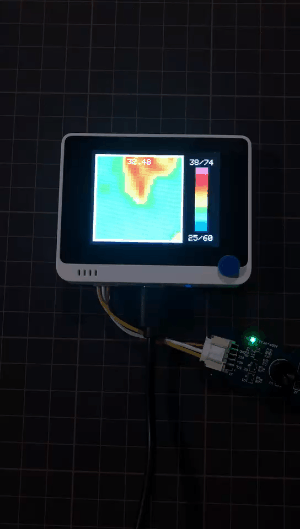### Getting Started by Raspberry Pi¶

#### Hardware¶

Materials required

Raspberry Pi 4 Grove Base Hat for Raspberry Pi Grove - Thermal Imaging Camera / IR Array MLX90641 110 degree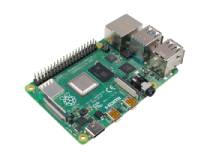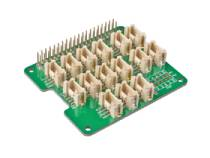Get one now Get one now Get one now

#### Hardware Connection¶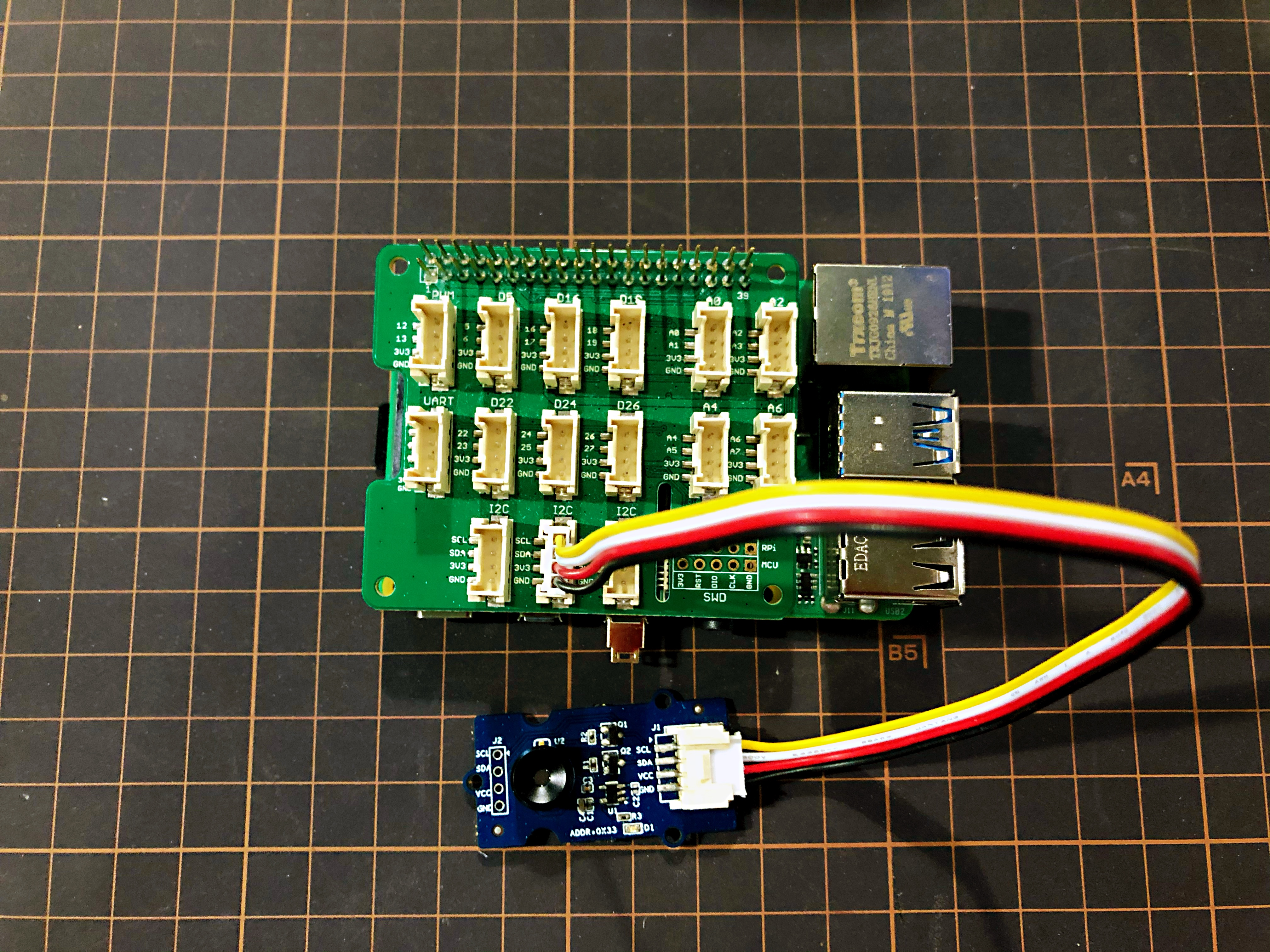• Step 1 Connect the Grove - Thermal Imaging Camera to one of the two I2C ports.
• Step 2 Plug the Raspberry Pi 4 into Grove Base Hat for Raspberry Pi.
• Step 3 Connect the Raspberry Pi to a display via HDMI cable, and power on the Raspberry Pi 4 by USB type-C.

#### Software¶

Raspberry Pi 4 supports Python, so the project demo can be easily displayed from the Raspberry Pi 4 display if you follow the below steps.

• Step 1 Install grove.py by the command
pip3 install Seeed-grove.py

• Step 2 Install the MLX90641 driver with the following command. Python environment(If you don't have authority of your Raspberry Pi):
pip3 install seeed-python-mlx9064x


pip3 install --upgrade seeed-python-mlx9064x

• Step 3 Check the corresponding i2c number of the Raspberry Pi:
ls /dev/i2c*


You may get the result like this:

/dev/i2c-1

• Step 4 Download the MLX90641 Library by git clone with command.

• Step 5 Run the BasicReadings.py file by the following commands: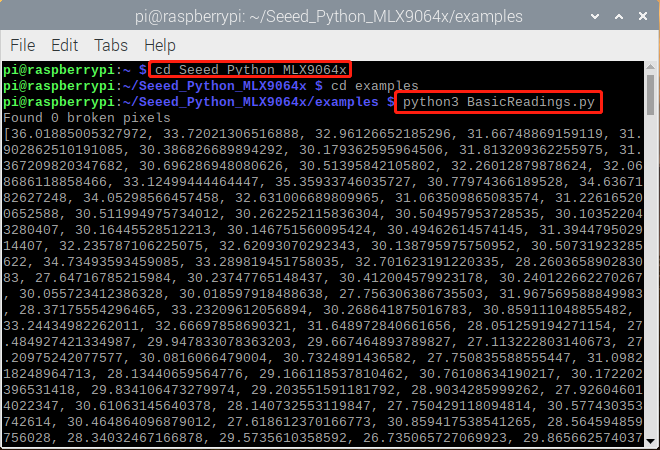Success

The outcome will be displayed as above if everything goes well.

Note

An upgrated UI of outcome on Raspberry Pi has been released as following:

• Step 1 Install pyqt5:
sudo apt-get install python3-pyqt5 -y

• Step 2 Installing from PyPI:
sudo pip3 install seeed_python_ircamera

• Step 3 Set the max i2c speed then reboot:
sudo sh -c "echo dtparam=i2c_arm=on,i2c_arm_baudrate=400000 >> /boot/config.txt"
sudo reboot

• Step 4 Input below command in terminal:
sudo ircamera I2C MLX90641


Success

The outcome will be displayed as following if everything goes well.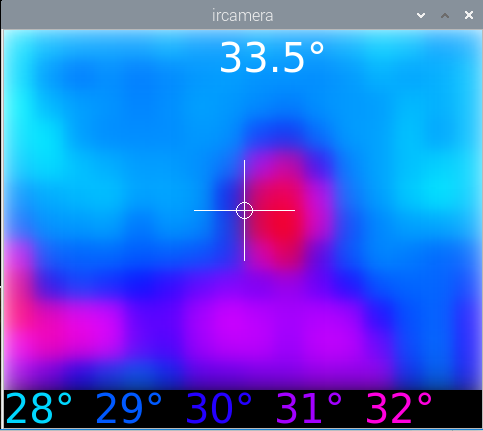## Tech Support¶

Please submit any technical issue into our forum.